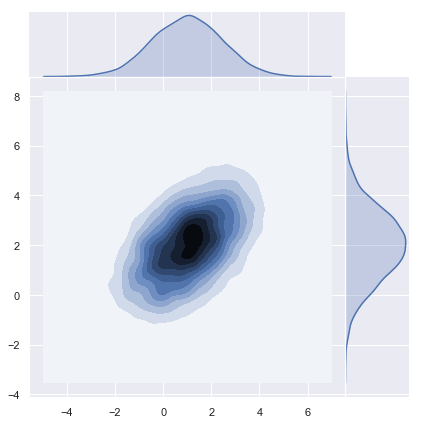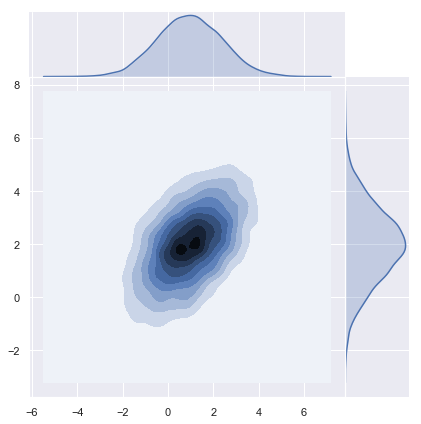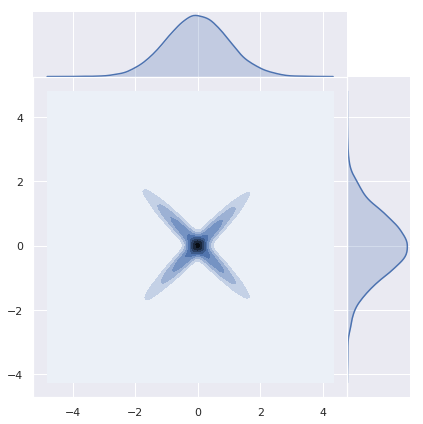# Sampling from a Multivariate Normal Distribution

In this post I want to describe how to sample from a multivariate normal distribution following section A.2 Gaussian Identities of the book Gaussian Processes for Machine Learning. This is a first step towards exploring and understanding Gaussian Processes methods in machine learning.

## Multivariate Normal Distribution

Recall that a random vector $$X = (X_1, , X_d)$$ has a multivariate normal (or Gaussian) distribution if every linear combination

$\sum_{i=1}^{d} a_iX_i, \quad a_i\in\mathbb{R}$ is normally distributed.

Warning: The sum of two normally distributed random variables does not need to be normally distributed (see below).

The multivariate normal distribution has a joint probability density given by

$p(x|m,K_0) =(2\pi)^{−d/2}|K_0|^{−1/2}\exp\left(-\frac{1}{2}(x−m)^T {K_0}^{-1}(x−m)\right),$

where $$m ^d$$ is the mean vector and $$K_0 M_d()$$ is the (symmetric, positive definite) covariance matrix.

## Prepare Notebook

import numpy as np
import matplotlib.pyplot as plt
import seaborn as sns; sns.set()

# Set Parameters

# Define dimension.
d = 2

# Set mean vector.
m = np.array([1, 2]).reshape(2, 1)

# Set covariance function.
K_0 = np.array([[2, 1],
[1, 2]])

Let us compute the eigenvalues of $$K_0$$.

# Eigenvalues covariance function.
np.linalg.eigvals(K_0)
array([3., 1.])

We see that $$K_0$$ is indeed positive definite (see The Spectral Theorem for Matrices).

## Sampling Process

### Step 1: Compute the Cholesky Decomposition

We want to compute the Cholesky decomposition of the covariance matrix $$K_0$$. That is, we want to find a lower triangular matrix $$LM_d()$$ such that $$K_0 = LL^T$$.

“In practice it may be necessary to add a small multiple of the identity matrix $$I$$ to the covariance matrix for numerical reasons. This is because the eigenvalues of the matrix $$K_0$$ can decay very rapidly and without this stabilization the Cholesky decomposition fails. The effect on the generated samples is to add additional independent noise of variance . From the context  can usually be chosen to have inconsequential effects on the samples, while ensuring numerical stability.” (A.2 Gaussian Identities)

# Define epsilon.
epsilon = 0.0001

K = K_0 + epsilon*np.identity(d)
#  Cholesky decomposition.
L = np.linalg.cholesky(K)
L
array([[1.41424892, 0.        ],
[0.7070891 , 1.2247959 ]])

Let us verify the desired property:

np.dot(L, np.transpose(L))
array([[2.0001, 1.    ],
[1.    , 2.0001]])

### Step 2: Generate Independent Samples $$u ∼ N(0,I)$$

# Number of samples.
n = 10000

u = np.random.normal(loc=0, scale=1, size=d*n).reshape(d, n)

### Step 3: Compute $$x = m + Lu$$

The variable $$x = m + Lu$$ has a multivariate normal distribution since is a linear combination of independent normally distributed variables. Moreover,

$E[x] = E[m + Lu] = m + LE[u] = m$

and

$E[xx^T] = E[mm^T] + E[mu^TL^T] + E[Lum^T] + E[Luu^TL^T] = ||m^2|| + LE[uu^T]L^T = \lVert m \rVert^2 + K$

hence, $$E[(x-m)(xT-mT)] = K$$.

x = m + np.dot(L, u)

## Plot Distribution

Let us plot the density function.

sns.jointplot(x=x, y=x, kind="kde", space=0);## Using Numpy Sampler

Numpy has a build in multivariate normal sampling function:

z = np.random.multivariate_normal(mean=m.reshape(d,), cov=K, size=n)
y = np.transpose(z)
# Plot density function.
sns.jointplot(x=y, y=y, kind="kde", space=0);## Sums of Normal Random Variables need not be Normal

As an important remark, note that sums of normal random variables need not be normal. Let us see a concrete example studied in detail here. Let $$Z_1 N(0,1)$$ and define $$Z_2 := (Z_1)Z_1$$. Then, $$Z_1 + Z_2$$ is not normally distributed.

z_1 = np.random.normal(loc=0, scale=1, size=n)
z = np.random.normal(loc=0, scale=1, size=n)
z_2 = np.sign(z)*z_1
sns.jointplot(x=z_1, y=z_2, kind="kde", space=0);